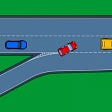# DP and binary search

`class Solution:    def maxSideLength(self, mat: List[List[int]], threshold: int) -> int:        R, C = len(mat), len(mat)        dp = [[0 for j in range(C+1)] for i in range(R+1)]        for i in range(R):            for j in range(C):                dp[i+1][j+1] = dp[i][j+1] + dp[i+1][j] - dp[i][j] + mat[i][j]        cur_max = 0        for i in range(R):            for j in range(C):                for L in range(cur_max+1, min(R,C)+1):                    if i+L-1<R and j+L-1<C and dp[i+L][j+L] - dp[i+L][j] - dp[i][j+L] + dp[i][j]  <= threshold:                        cur_max = L        return cur_max if cur_max!=1 else 0`
`class Solution:    def maxSideLength(self, mat: List[List[int]], threshold: int) -> int:        R, C = len(mat), len(mat)        dp = [[0 for j in range(C+1)] for i in range(R+1)]        for i in range(R):            for j in range(C):                dp[i+1][j+1] = dp[i][j+1] + dp[i+1][j] - dp[i][j] + mat[i][j]        cur_max = 0        def binary(i, j, l, r, cur_max):            if l>r or i+l>R or j+l>C or dp[i+l][j+l] - dp[i+l][j] - dp[i][j+l] + dp[i][j] > threshold:return cur_max            while l<r:                m = (l+r+1)//2                if i+m>R or j+m>C or dp[i+m][j+m] - dp[i+m][j] - dp[i][j+m] + dp[i][j] > threshold:                    r = m - 1                else:                    l = m            return l        for i in range(R):            for j in range(C):                cur_max = binary(i, j, cur_max, min(R,C)+1, cur_max)        return cur_max if cur_max!=1 else 0`

# 887. Super Egg Drop

• The number of eggs left to throw `i`, (0 <= i <= K)
• The number of floors left to test `j`, (1 <= j <= N)

# DP formula

• base case
• recursive relation
`dp[j] = j, j = 1...N # one egg, check each floor from 1 to jdp[i] = 0, i = 1...K # no floor, no drop needed to get the optimal floordp[i] = 1, i = 1...K # one floor, only check once`
• the egg brokes, I now have 2 eggs, and the floor to choose becomes 1~24.
• the egg remains safe, I still have 3 eggs, and the floor to choose becomes 26~100.

# The brute force solution

`from functools import lru_cacheclass Solution:    def superEggDrop(self, K: int, N: int) -> int:        @lru_cache(None)        def dp(k, n):            if k == 1:return n            if k == 0:return float('inf')            if n == 0:return 0            if n == 1:return 1            return min( max(1+dp(k-1, i-1), 1+dp(k, n-i)) for i in range(1, n+1))        return dp(K, N)`
`from functools import lru_cacheclass Solution:    def superEggDrop(self, K: int, N: int) -> int:        @lru_cache(None)        def dp(k, n):            if k == 1:return n            if k == 0:return float('inf')            if n == 0:return 0            if n == 1:return 1            def binary(k, n):                l, r = 1, n                while l<r:                    m = (l+r+1)//2                    left, right = dp(k-1, m-1), dp(k, n-m)                    if left > right:                        r = m -1                    else:                        l = m                     return 1+max(dp(k-1, l-1), dp(k, n-l))            return binary(k, n)        return dp(K, N)`
`class Solution:    def superEggDrop(self, K: int, N: int) -> int:        dp = [ * (K + 1) for i in range(N + 1)]        for m in range(1, N + 1):            for k in range(1, K + 1):                dp[m][k] = dp[m - 1][k - 1] + dp[m - 1][k] + 1            if dp[m][K] >= N: return m`

--

--

--

## More from Jimmy Shen

Data Scientist/MLE/SWE @takemobi

Love podcasts or audiobooks? Learn on the go with our new app.

## 4 Easy Ways To Beat “ Fizz Buzz” In Python## MATERIALIZED VIEW vs VIEW in PostgreSQL## Naming your functions, files & paths## Adding Time Lag to Monitor Kafka Consumer## As a programmer, do you think beginners are getting obsessed with data structures and algorithms?## Jimmy Shen

Data Scientist/MLE/SWE @takemobi

## Leetcode 534 Game Play Analysis III without sum window function## Merge k Sorted Lists using minheap in O(N logK) time